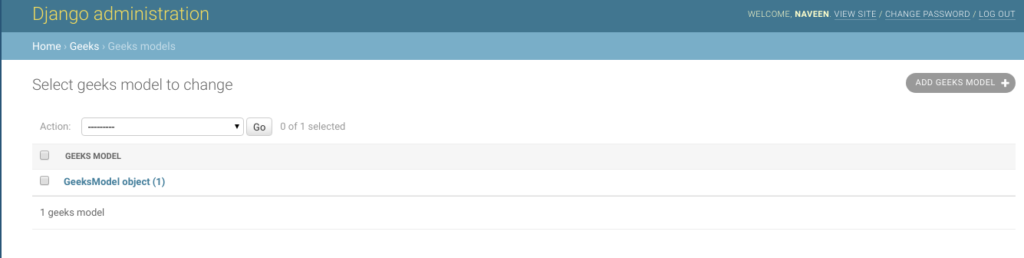# BooleanField – Django Models

• Last Updated : 24 Aug, 2021

BooleanField is a true/false field. It is like a bool field in C/C+++. The default form widget for this field is CheckboxInput, or NullBooleanSelect if null=True. The default value of BooleanField is None when Field.default isn’t defined. One can define the default value as true or false by setting the default attribute to true/false simultaneously.

Syntax:

`field_name = models.BooleanField(**options)`

## Django Model BooleanField Explanation

Illustration of BooleanField using an Example. Consider a project named geeksforgeeks having an app named geeks.

Refer to the following articles to check how to create a project and an app in Django.

Enter the following code into models.py file of geeks app.

## Python3

 `from` `django.db ``import` `models``from` `django.db.models ``import` `Model``# Create your models here.` `class` `GeeksModel(Model):``    ``geeks_field ``=` `models.BooleanField()`

Add the geeks app to INSTALLED_APPS

## Python3

 `# Application definition` `INSTALLED_APPS ``=` `[``    ``'django.contrib.admin'``,``    ``'django.contrib.auth'``,``    ``'django.contrib.contenttypes'``,``    ``'django.contrib.sessions'``,``    ``'django.contrib.messages'``,``    ``'django.contrib.staticfiles'``,``    ``'geeks'``,``]`

Now when we run makemigrations command from the terminal,

`Python manage.py makemigrations`

A new folder named migrations would be created in geeks directory with a file named 0001_initial.py

## Python3

 `# Generated by Django 2.2.5 on 2019-09-25 06:00` `from` `django.db ``import` `migrations, models` `class` `Migration(migrations.Migration):` `    ``initial ``=` `True` `    ``dependencies ``=` `[``    ``]` `    ``operations ``=` `[``        ``migrations.CreateModel(``            ``name ``=``'GeeksModel'``,``            ``fields ``=``[``                ``(``'id'``,``                  ``models.AutoField(auto_created ``=` `True``,``                  ``primary_key ``=` `True``,``                  ``serialize ``=` `False``,``                  ``verbose_name ``=``'ID'``                ``)),``                ``(``'geeks_field'``, models.BooleanField()),``            ``],``        ``),``    ``]`

Now run,

`Python manage.py migrate`

Thus, an geeks_field BooleanField is created when you run migrations on the project. It is a field to store boolean value – True/False.

## How to use BooleanField ?

BooleanField is used for checking the particular condition, for example, to check if Email of a user is verified or not. One can use BooleanField to mark it True or False. We can also set it to False by using default=True.

## Python3

 `# importing the model``# from geeks app``from` `geeks.models ``import` `GeeksModel` `# creating a instance of``# GeeksModel``geek_object ``=` `GeeksModel.objects.create(geeks_field ``=` `True``)``geek_object.save()`

Now let’s check it in admin server. We have created an instance of GeeksModel.### Field Options

Field Options are the arguments given to each field for applying some constraint or imparting a particular characteristic to a particular Field. For example, adding an argument null = True to BooleanField will enable it to store empty values for that table in a relational database.
Here are the field options and attributes that a BooleanField can use.

My Personal Notes arrow_drop_up